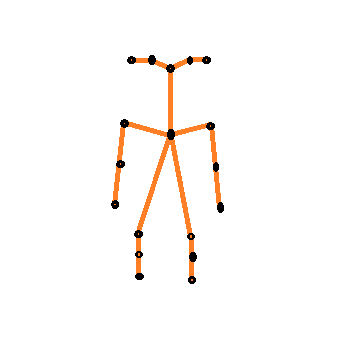# QPainterPath::toFillPolygon() on an open polygon returns wrong values

• I have been trying to draw skeletal points of a human using 18 different joints. So, i decided to use `QGraphicsPathItem`. I could successfully generate the item which looks something like this: (pardon my drawing skills)To achieve this i used:

``````QPainterPath pp;

pp.moveTo(m_points);
pp.lineTo(m_points);
pp.lineTo(m_points);
pp.lineTo(m_points);
pp.lineTo(m_points);

pp.moveTo(m_points);
pp.lineTo(m_points);
pp.lineTo(m_points);
pp.lineTo(m_points);

pp.moveTo(m_points);
pp.lineTo(m_points);
pp.lineTo(m_points);
pp.lineTo(m_points);

pp.moveTo(m_points);
pp.lineTo(m_points);
pp.lineTo(m_points);
pp.lineTo(m_points);

pp.moveTo(m_points);
pp.lineTo(m_points);
pp.lineTo(m_points);

pp.moveTo(m_points);
pp.lineTo(m_points);
pp.lineTo(m_points);

m_item->setPath(pp);
``````

At some point of time when i wish to know the positions of the points, i use:

``````QPolygonF polygon = m_item->path().toFillPolygon();
``````

this returns me 33 points instead of 18.

I feel this is because my polygon is open (with no same start and end point). I tested `toFillPolygon()` on a closed polygon it works fine.

Is there a way to get the current positions of those 18 points i started with from the `path()`? Or how do we extract all the points in an open polygon path?

• After realizing i cannot get points from `toFillPolygon()` i stumbled on this link. It helped me understand we can not extract points from `QPainterPath` using `toFillPolygon()` when our path itself is OPEN POLYGON i.e. starting and ending points are different. So, i used something like this:

``````QVector<QPointF> pointsTable;
QPainterPath path = m_item->path();

pointsTable.reserve(18);

// push the point 0 manually
// since it is created using moveTo()
// but we need this starting point
pointsTable.push_back(mapToScene(path.elementAt(0)));

// iterate through all the elements of the path
for (int idx = 0; idx < path.elementCount(); ++idx)
{
// DO NOT consider moveTo() elements
// we only need lineTo() elements
if (!path.elementAt(idx).isMoveTo())
{
// push into the container
pointsTable.push_back(mapToScene(path.elementAt(idx)));
}
}
``````

Notice i do not consider the elements `moveTo()` but only the elements `lineTo()` except for the first `moveTo()` element which is my starting position.

So, i can get current position of all the 18 points i initially started with to draw the path.

• Hi
Did you try with https://doc.qt.io/qt-5/qpainterpath.html#toSubpathPolygons

The reason you might not get any answers yet
is that 11 hours is not long enough as users are in different time zones.

• After realizing i cannot get points from `toFillPolygon()` i stumbled on this link. It helped me understand we can not extract points from `QPainterPath` using `toFillPolygon()` when our path itself is OPEN POLYGON i.e. starting and ending points are different. So, i used something like this:

``````QVector<QPointF> pointsTable;
QPainterPath path = m_item->path();

pointsTable.reserve(18);

// push the point 0 manually
// since it is created using moveTo()
// but we need this starting point
pointsTable.push_back(mapToScene(path.elementAt(0)));

// iterate through all the elements of the path
for (int idx = 0; idx < path.elementCount(); ++idx)
{
// DO NOT consider moveTo() elements
// we only need lineTo() elements
if (!path.elementAt(idx).isMoveTo())
{
// push into the container
pointsTable.push_back(mapToScene(path.elementAt(idx)));
}
}
``````

Notice i do not consider the elements `moveTo()` but only the elements `lineTo()` except for the first `moveTo()` element which is my starting position.

So, i can get current position of all the 18 points i initially started with to draw the path.